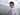# 31. 下一个排列

April 15, 2020

https://leetcode-cn.com/problems/next-permutation/

# 解：

``````func nextPermutation(nums []int)  {
if len(nums) <= 1 {
return
}

i := len(nums) - 2
for ; i >= 0; i-- {
if nums[i] < nums[i+1] {
j := i + 1
for j + 1 < len(nums) {
if nums[i] >= nums[j + 1] {
break
}
j++
}
nums[i], nums[j] = nums[j], nums[i]
break
}
}
k := len(nums) - 1
i++
for i < k {
nums[i], nums[k] = nums[k], nums[i]
i++
k--
}
}``````